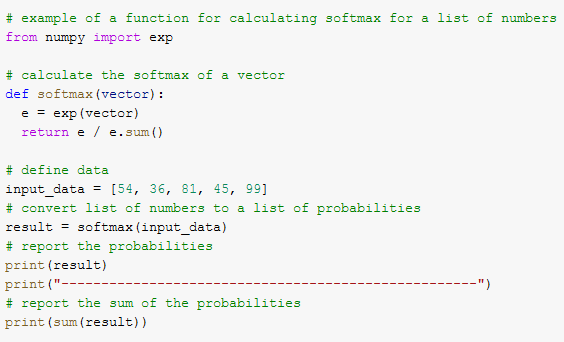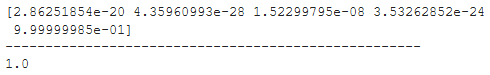# Keras Activation Layers – Ultimate Guide for Beginners

## Introduction

Activation functions are an integral part of neural networks in Deep Learning and there are plenty of them with their own use cases. In this article, we will understand what is Keras activation layer and its various types along with syntax and examples. We will also learn about the advantages and disadvantages of each of these Keras activation functions. But before going there, let us first understand briefly what is activation function in Neural Networks.

### What is an Activation Function?

The idea of activation functions is derived from the neuron-based model of the human brain. Brains consist of a complex network of biological neurons in which a neuron is activated based on certain input from the previous neuron. A series of such activation of neurons enables body functions.

In the artificial neural network, we have artificial neurons which are nothing but a mathematical unit consisting of the activation function. It also fires the output based on certain inputs received by the previous neuron.

The below diagram explains the analogy between the biological neuron and artificial neuron.

### Characteristics of good Activation Functions in Neural Network

There are many activation functions that can be used in neural networks. Before we take a look at the popular ones in Kera let us understand what is an ideal activation function.

• Non-Linearity – Activation function should be able to add nonlinearity in neural networks especially in the neurons of hidden layers. This is because almost all real-world scenarios can be explained with a non-linear relationship only, they are hardly linear.
• Differentiable – The activation function should be differentiable. During the training phase, the neural network learns by back-propagating error from the output layer to hidden layers. The backpropagation algorithm uses the derivative of the activation function of neurons in hidden layers, to adjust their weights so that error in the next training epoch can be reduced.
In :
```import tensorflow as tf
```

## Types of Activation Layers in Keras

Now in this section, we will learn about different types of activation layers available in Keras along with examples and pros and cons.

## 1. Relu Activation Layer

ReLu Layer in Keras is used for applying the rectified linear unit activation function.

#### Advantages of ReLU Activation Function

• ReLu activation function is computationally efficient hence it enables neural networks to converge faster during the training phase.
• It is both non-linear and differentiable which are good characteristics for activation function.
• ReLU does not suffer from the issue of Vanishing Gradient issue like other activation functions and hence it is very effective in hidden layers of large neural networks.

#### Disadvantages of ReLU Activation Function

• The major disadvantage of the ReLU layer is that it suffers from the problem of Dying Neurons. Whenever the inputs are negative, its derivative becomes zero, therefore backpropagation cannot be performed and learning may not take place for that neuron and it dies out.

Syntax of ReLu Activation Layer in Keras –

``````tf.keras.activations.relu(x, alpha=0.0, max_value=None, threshold=0)
``````

#### Example of ReLu Activation Layer in Keras

In :
```foo = tf.constant([-5, -8, 0.0, 3, 9], dtype = tf.float32)

tf.keras.activations.relu(foo).numpy()

tf.keras.activations.relu(foo, alpha=0.7).numpy()

tf.keras.activations.relu(foo, max_value=3).numpy()

tf.keras.activations.relu(foo, threshold=7).numpy()
```
Output:
`array([-0., -0.,  0.,  0.,  9.], dtype=float32)`

## 2. Sigmoid Activation Layer

In the Sigmoid Activation layer of Keras, we apply the sigmoid function. The formula of Sigmoid function is as below –

``````sigmoid(x) = 1/ (1 + exp(-x))
``````

The sigmoid activation function produces results in the range of 0 to 1 which is interpreted as the probability.

#### Advantages of Sigmoid Activation Function

• The sigmoid activation function is both non-linear and differentiable which are good characteristics for activation function.
• Since its output ranges from 0 to 1, it is a good choice for the output layer to produce the result in probability for binary classification.

#### Disadvantages of Sigmoid Activation Function

• Sigmoid activation is computationally heavy to use and the neural network may not converge fast during training.
• If the input values are too small or too high, then the neural network may stop learning, this problem is popularly known as the vanishing gradient problem. This is why the Sigmoid activation function is not used in hidden layers.

Syntax of Sigmoid Activation Layer in Keras

``````tf.keras.activations.sigmoid(x)
``````

#### Example of Sigmoid Activation Layer in Keras

In :
```a = tf.constant([-35, -5.0, 0.0, 6.0, 25], dtype = tf.float32)
b = tf.keras.activations.sigmoid(a)
b.numpy()
```
Output:
```array([6.3051174e-16, 6.6929162e-03, 5.0000000e-01, 9.9752736e-01,
1.0000000e+00], dtype=float32)```

## 3. Softmax Activation Layer

The softmax activation layer in Keras is used to implement Softmax activation in the neural network.

Softmax function produces a probability distribution as a vector whose value range between (0,1) and the sum equals 1.

#### Advantages of Softmax Activation Function

• Since Softmax produces a probability distribution, it is used as an output layer for multiclass classification.

Syntax of Softmax Activation Function in Keras

``````tf.keras.activations.softmax(x, axis=-1)
``````

#### Example of Softmax Activation Function

In :Output:## 4. Tanh Activation Function

Tanh Activation Layer in Keras is used to implement Tanh activation function for neural networks.

#### Advantages of Tanh Activation Function

• The Tanh activation function is both non-linear and differentiable which are good characteristics for activation function.
• Since its output ranges from -1 to +1, it can be used when we want the output of a neuron to be negative.

• Since its functioning is similar to a sigmoid function, it also suffers from the issue of Vanishing gradient if there are too low or too high input values.

Syntax of Tanh Activation Layer in Keras

``tf.keras.activations.tanh(x)``

#### Example of Tanh Activation Layer

In :
```a = tf.constant([5.0,-1.0, -5.0,6.0,3.0], dtype = tf.float32)
b = tf.keras.activations.tanh(a)
b.numpy()
```
Output:
```array([ 0.99990916, -0.7615942 , -0.99990916,  0.9999876 ,  0.9950547 ],
dtype=float32)```

## Which Activation Function to use in Neural Network?

• Sigmoid and Tanh activation function should be avoided in hidden layers as they suffer from Vanishing Gradient problem.
• Sigmoid activation function should be used in the output layer in case of Binary Classification
• ReLU activation functions are ideal for hidden layers of neural networks as they do not suffer from the Vanishing Gradient problem and are computationally fast.
• Softmax activation function should be used in the output layer in case of multiclass classification.

## Conclusion

This is the end of this Keras tutorial that talked about different activation functions in Keras. We also looked at the syntax and examples of activation functions namely ReLu, Sigmoid, Softmax, and Tanh along with a detailed comparison between them.

Reference Keras Documentation